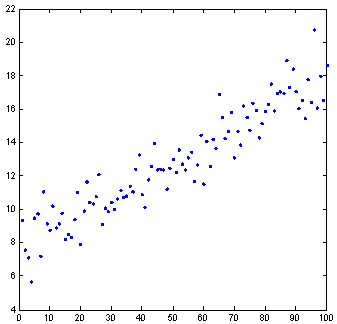# Averaging Raindrops - an exercise in geometric probability

### Scott E. Brodie 11/7/2010

Most everyone has had the experience of looking at a concrete sidewalk just as it starts to rain. As each raindrop produces a dark dot on the pavement, the random distribution of the falling raindrops becomes apparent - quite different from a regular grid. Here are some examples showing the first 100 drops:The random pattern of drops reflects three important features:

1. The distribution of drops is "uniform" -- any two disjoint regions of equal area within the square are equally likely to contain any chosen number of drops. Thus, we can use the area of a patch of sidewalk as a measure of the probability that a drop will land there. (If the area of our square of pavement is not equal to 1, we can divide by the area of the square to convert the raw area measure into a "probability measure," in which the total measure of the region is 1.)

2. The x- and y-coordinates of drops are "independent" - knowing the x-coordinate of a raindrop does not enhance the ability to predict the y-coordinate. This guarantees, for example, that the drops do not cluster along a particular line on the sidewalk, as shown in the next example:1. The location of each random drop is "independent" of the location of the others. This explains why the pattern of drops is "clumpy" or "blotchy." Indeed, the only way for the pattern to be more even would be for successive drops to "know" where the previous drops had landed so they could avoid landing nearby. The independence property assures us that our estimates of the number of drops in a patch of pavement based on its area will not be confounded by any structure or pattern in the distribution of the drops.

Suppose we restrict our attention to drops which fall within the "unit square" - that is, with x-coordinates and y-coordinates between 0 and 1. The blotchy nature of random events notwithstanding, we can still answer simple questions about the random pattern of raindrops. For example, what is the average of the x-coordinate of the raindrops? Because the x- and y-coordinates are independent, we can simply ignore the y-coordinate. The answer is obviously 1/2, as the x-coordinates fall uniformly on the interval from 0 to 1. Of course, the same reasoning indicates that the average value of the y-coordinate is also 1/2.

We may even ask a more subtle question. Say, what is the average value of the minimum of the x- and y- coordinates of the raindrops?

Since the distribution of raindrops is uniform, our question is equivalent to asking what is the average value of the function min(x, y) where x and y range over the interval from 0 to 1?

It is helpful to consider a three-dimensional plot of min(x, y) over this region:The surface min(x, y) is simply an asymmetrical pyramid with square base, rising to a vertex at the point (1, 1, 1). We seek the average height of this surface, or, equivalently the volume of the pyramid. (Of course, the average is the volume divided by the area of the base, but as the base is a square of side 1, the area of the base is simply 1.)

The formula for the volume of a pyramid is not nearly as well known as the formulas for more familiar volumes of prisms and spheres. In fact, the general case for pyramids of arbitrary shape cannot be obtained by the familiar methods of cutting-and-pasting which suffice for determining the area of, say, an arbitrary triangle. However, for certain pyramids, including this one, we can compute the volume by elementary means.

Consider a cube of edge 2 units, whose faces are 2×2 squares. Place a point at the center of the cube, and connect this central point to the 8 corners of the cube:These diagonal lines define six equal square-based pyramids, each with volume 1/6 of the volume of the cube (which is 2³ = 8).Our asymmetric pyramid occupies one quarter of the "bottom" pyramid in the cube, so its volume is

V = 8 × (1/6) × (1/4) = 8/24 = 1/3

We thus conclude that, over the region where x and y range independently from 0 to 1,

Average[min(x, y)] = 1/3.### Geometric Probability